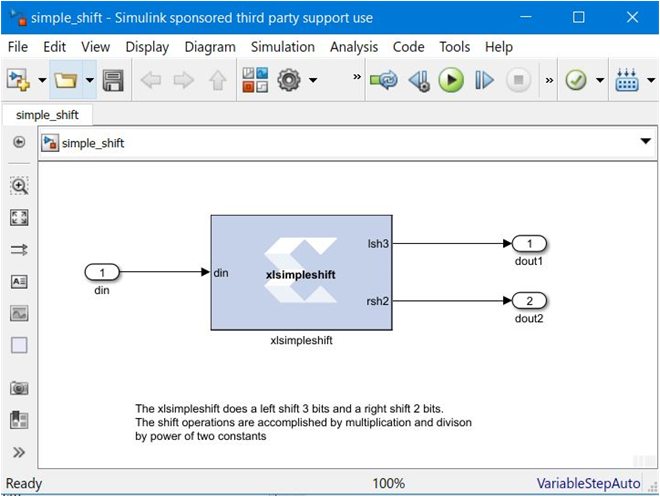# Shift Operations - 2022.1 English

## Vitis Model Composer User Guide (UG1483)

Document ID
UG1483
Release Date
2022-05-26
Version
2022.1 English

This example shows how to implement bit-shift operations using the MCode block. Shift operations are accomplished with multiplication and division by powers of two. For example, multiplying by 4 is equivalent to a 2-bit left-shift, and dividing by 8 is equivalent to a 3-bit right-shift. Shift operations are implemented by moving the binary point position and if necessary, expanding the bit width. Consequently, multiplying a Fix_8_4 number by 4 results in a Fix_8_2 number, and multiplying a Fix_8_4 number by 64 results in a Fix_10_0 number.

The following shows the `xlsimpleshift.m` file which specifies one left-shift and one right-shift:

``````function [lsh3, rsh2] = xlsimpleshift(din)
% [lsh3, rsh2] = xlsimpleshift(din) does a left shift
% 3 bits and a right shift 2 bits.
% The shift operation is accomplished by
% multiplication and division of power
% of two constant.
lsh3 = din * 8;
rsh2 = din / 4;
``````

The following diagram shows the subsystem after compilation:

Figure 1. Shift Operations